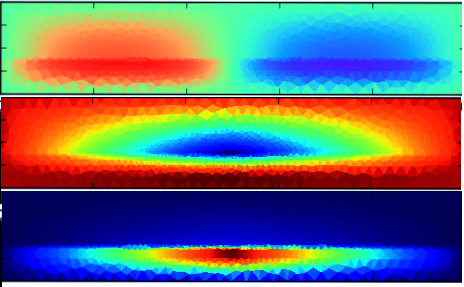#Multi-Point Stress Approximation (MPSA)
This module implements a discretization of poro-mechanics by cell centered finite volume methods.

The module provides discretization methods for three different equations:

1. Scalar elliptic equations (Darcy flow), using multipoint flux approximations (this is more or less equivalent to the MPFA implementation in the MRST's `mpfa` module, although the implementation and data structures is slightly different).
2. Linear elasticity, using Multipoint stress approximations.
3. Poro-mechanics, e.g., coupling terms for the combined system of 1. and 2.

Examples of usage for the three options can be found in `mpfa_ex`, `mpsa_ex`, and `biot_ex`, respectively, see the `examples` folder. The code has been tested for 2D and 3D problems on Cartesian and simplex grids, see papers below for illustrations.Displacements (upper and middle) and pressure increase under injection in a layered formation.

This implementation uses the weakly symmetric formulation used in  (specifically using vertex-based averaging).

Further information on the methods implemented herein, in particular the discretization of elasticity and poro-mechanics, can be found in the following papers (all open access)

## References

1. J. M. Nordbotten: Stable cell-center finite volume disrcetizaiton for Biot equations - SIAM J. Numer. Anal. 54(2) 942-968.
2. J. M. Nordbotten: Convergence of a cell-centered finite volume discretization for linear elasticity - SIAM J. Numer. Anal. 53(2) 2605-2625.
3. E. Keilegavlen, J. M. Nordbotten: Finite volume methods for elasticity with weak symmetry, arXiv:1512.01042

Published November 25, 2016